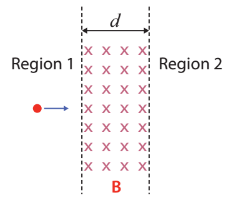Physics Practice Problems Circular Motion of Charges in Magnetic Fields Practice Problems Solution: Two regions in space, Region 1 and Region 2, are s...

🤓 Based on our data, we think this question is relevant for Professor Saab's class at UF.

# Solution: Two regions in space, Region 1 and Region 2, are separated by a gap of thickness  d filled with a magnetic field of B pointing into the page, as shown in the figure.  If d = 1 cm and B = 0.1 T, what is the minimum kinetic energy required for an electron to penetrate from Region 1 into Region 2? (1) 1.4 × 10−14 J (2) 2.8 × 10−14 J (3) 2.1 × 10−14 J (4) 3.5 × 10−14 J (5) 4.2 × 10−14 J

###### Problem

Two regions in space, Region 1 and Region 2, are separated by a gap of thickness  d filled with a magnetic field of B pointing into the page, as shown in the figure.  If d = 1 cm and B = 0.1 T, what is the minimum kinetic energy required for an electron to penetrate from Region 1 into Region 2?

(1) 1.4 × 10−14 J

(2) 2.8 × 10−14 J

(3) 2.1 × 10−14 J

(4) 3.5 × 10−14 J

(5) 4.2 × 10−14 JCircular Motion of Charges in Magnetic Fields

Circular Motion of Charges in Magnetic Fields

#### Q. Consider the circular motion of a positively charged particle in the plane of this paper, due to a constant magnetic field B which points out of the p...

Solved • Mon Jun 26 2017 12:23:28 GMT-0400 (EDT)

Circular Motion of Charges in Magnetic Fields

#### Q. A static uniform magnetic field is directed into the page. A charged particle moves in the plane of the page following a counterclockwise spiral of de...

Solved • Mon May 01 2017 13:39:39 GMT-0400 (EDT)

Circular Motion of Charges in Magnetic Fields

#### Q. In the figure, a uniform magnetic field points into the page. (The magnetic field vectors are indicated by ⊗ symbols.). Four particles with the same m...

Solved • Fri Apr 07 2017 12:18:57 GMT-0400 (EDT)

Circular Motion of Charges in Magnetic Fields

#### Q. A small sphere with positive charge q = 5.00 x 10 -4 C and mass 2.00 x 10 -5 kg is moving in a region of uniform magnetic field. The object moves coun...

Solved • Thu Jan 26 2017 13:46:02 GMT-0500 (EST)